# RS Aggarwal Solutions for Class 7 Maths Exercise 20D Chapter 20 Mensuration

The simple PDF of RS Aggarwal Solutions for the Exercise 20D of Class 7 Maths Chapter 20, Mensuration is the best study material for those students who are finding complications in solving problems. Areas of the triangle are the only one topic that is covered in this RS Aggarwal Solutions for Class 7 Maths Chapter 20 Mensuration. Our expert professors formulate these exercises to assist you with your exam preparation to score good marks in Maths. Students who wish to score good marks in Maths practise RS Aggarwal Class 7 Solutions Chapter 20.

## Download the PDF of RS Aggarwal Solutions For Class 7 Maths Chapter 20 Mensuration – Exercise 20D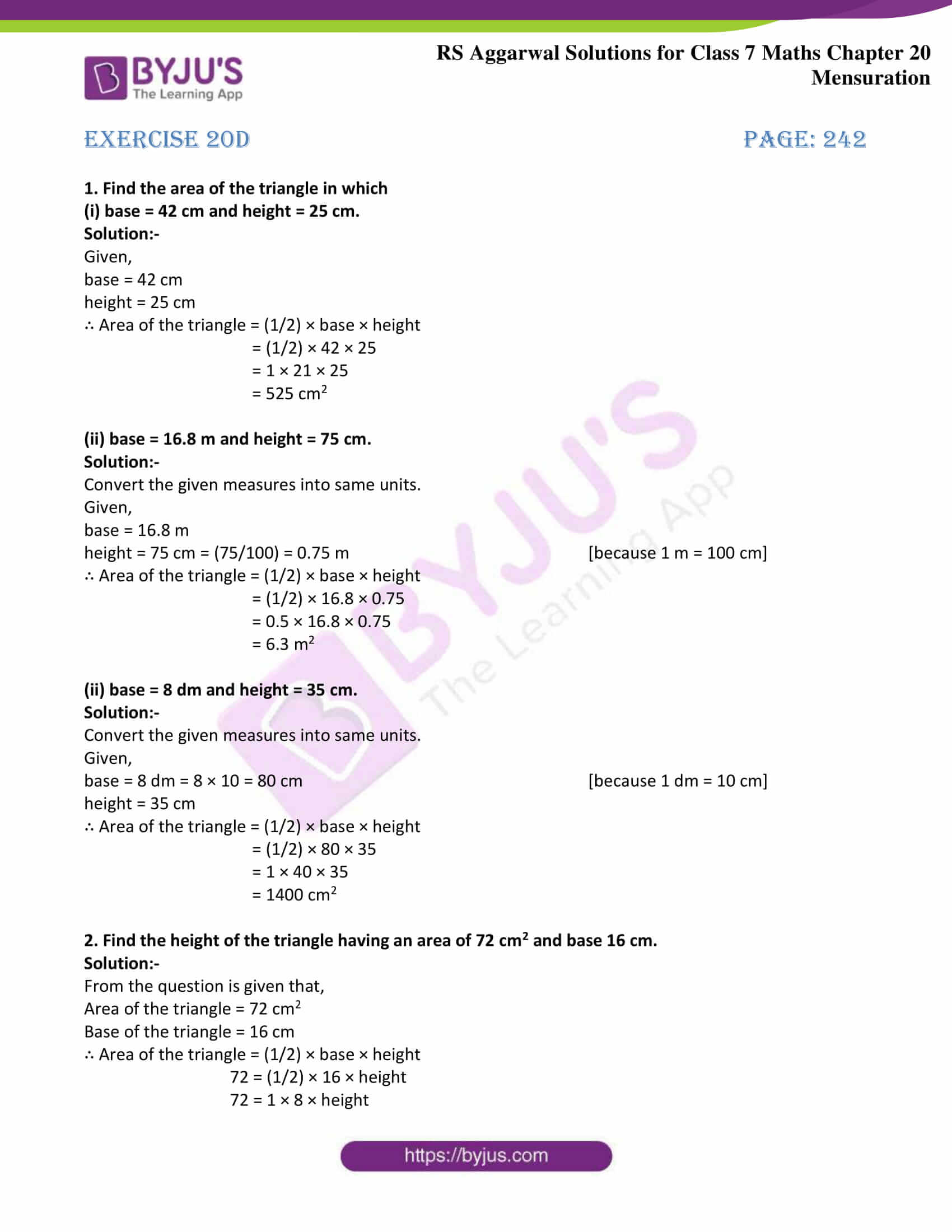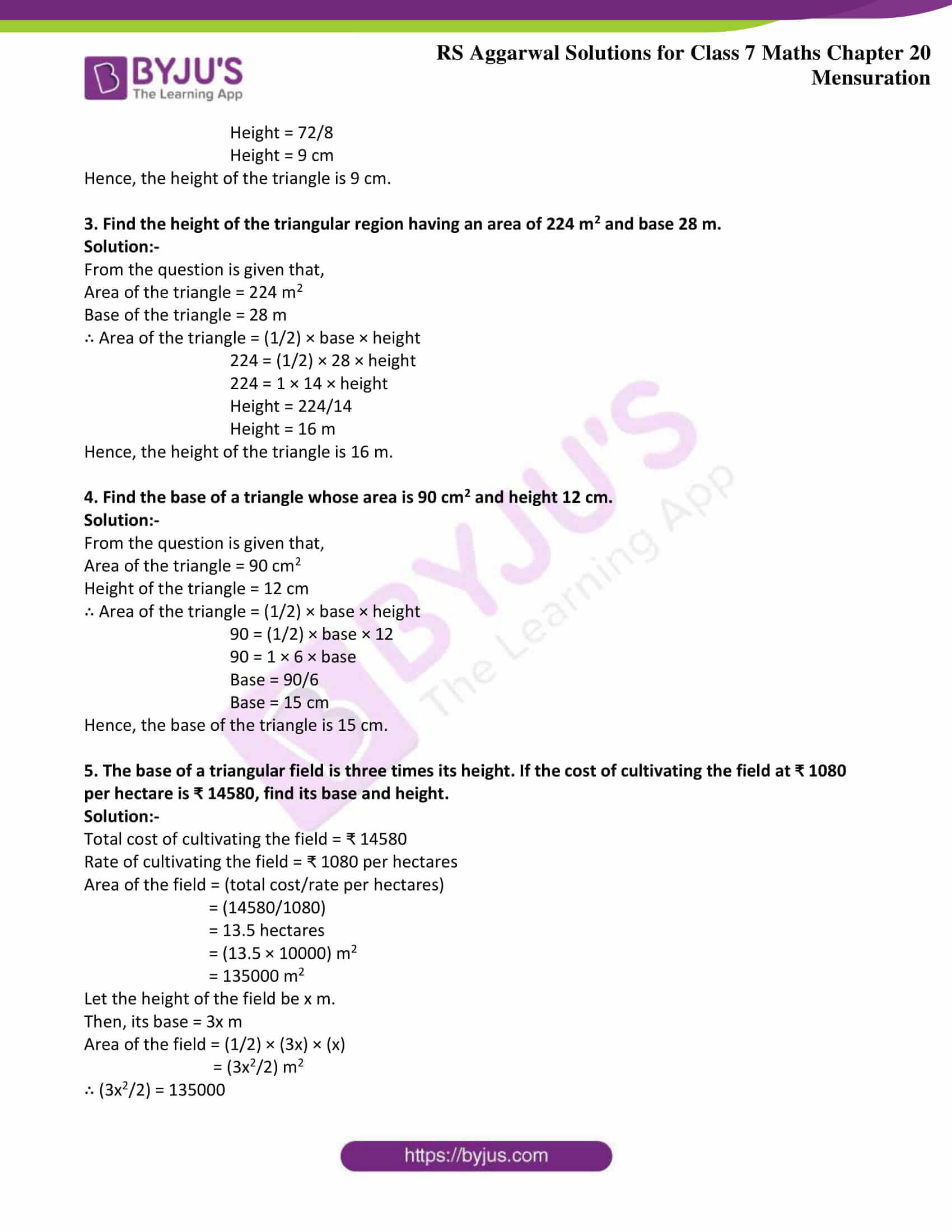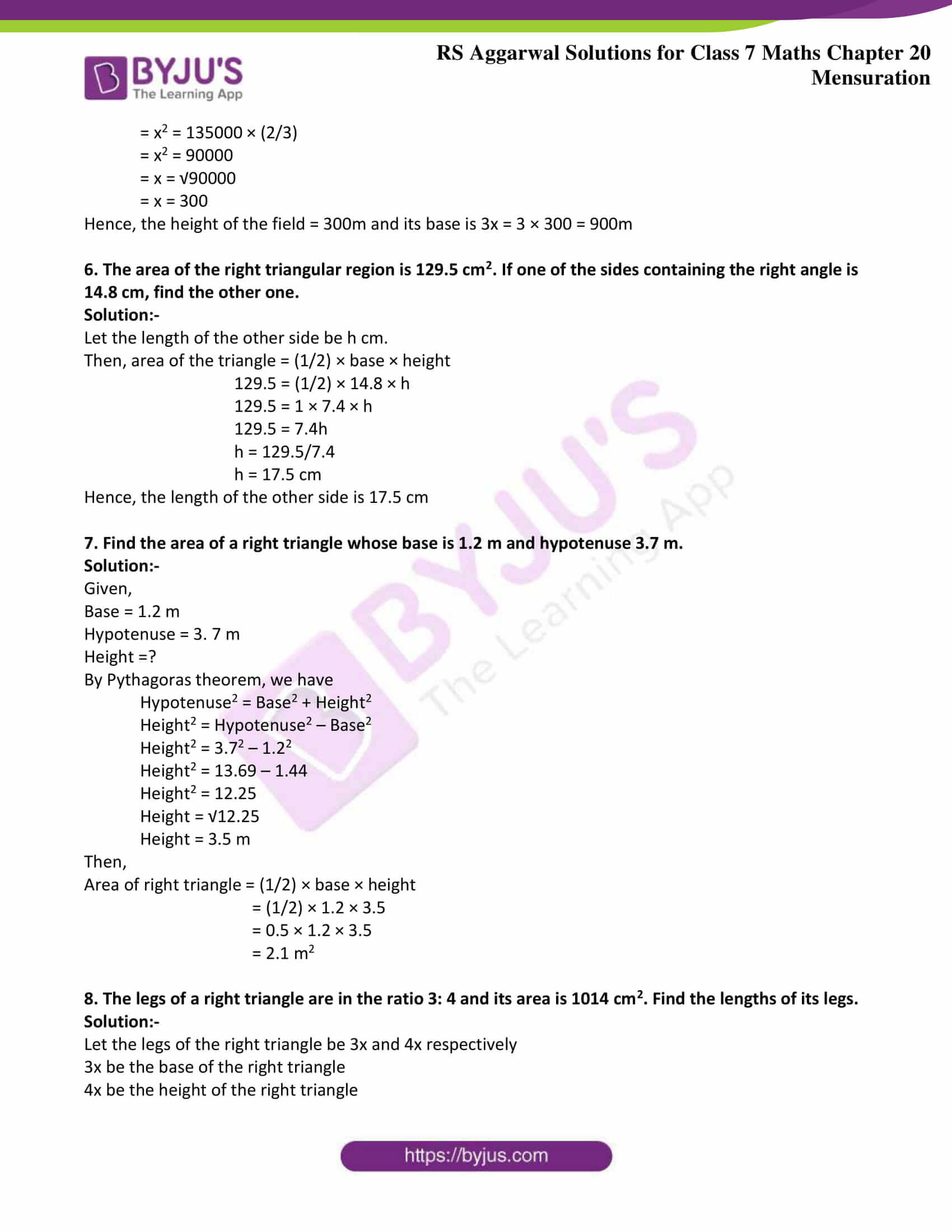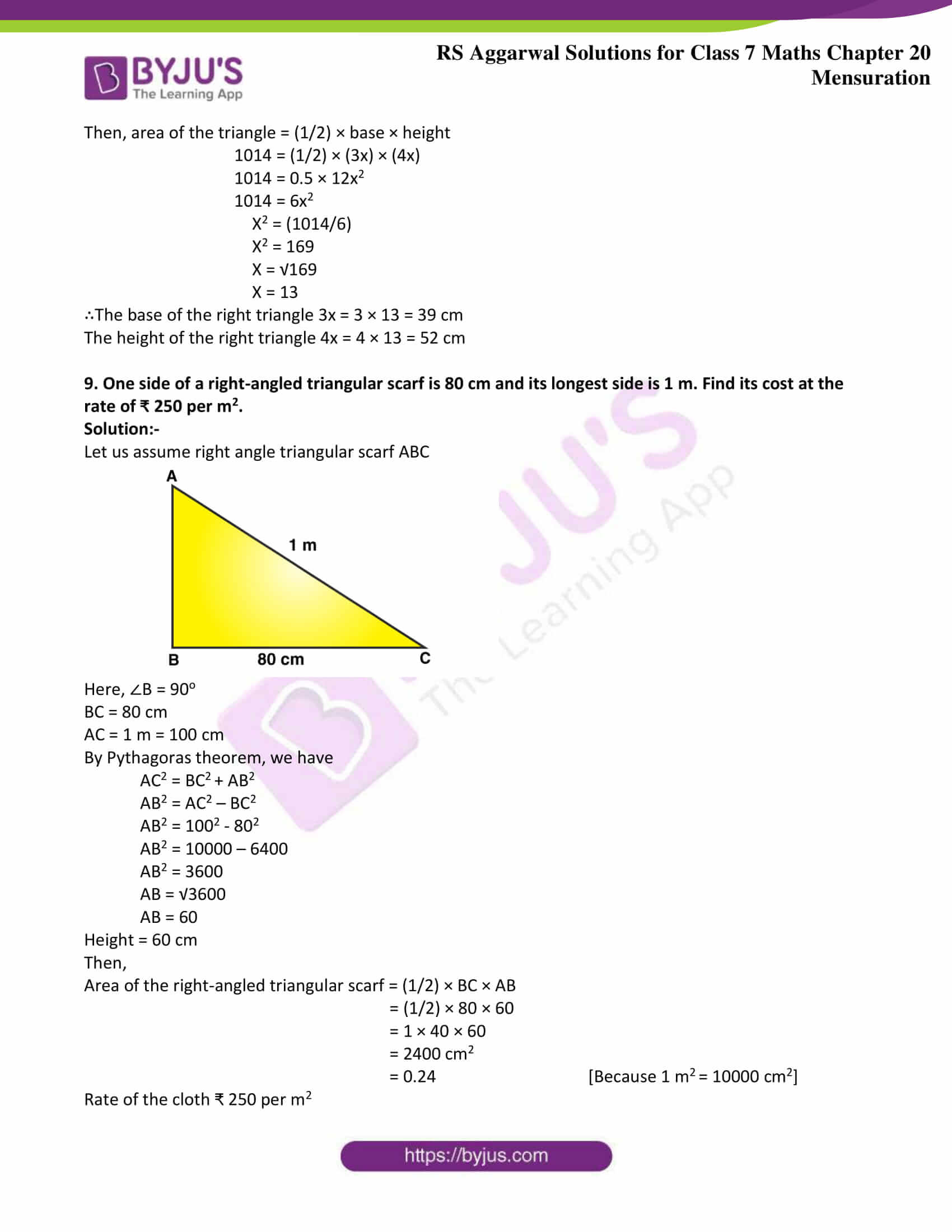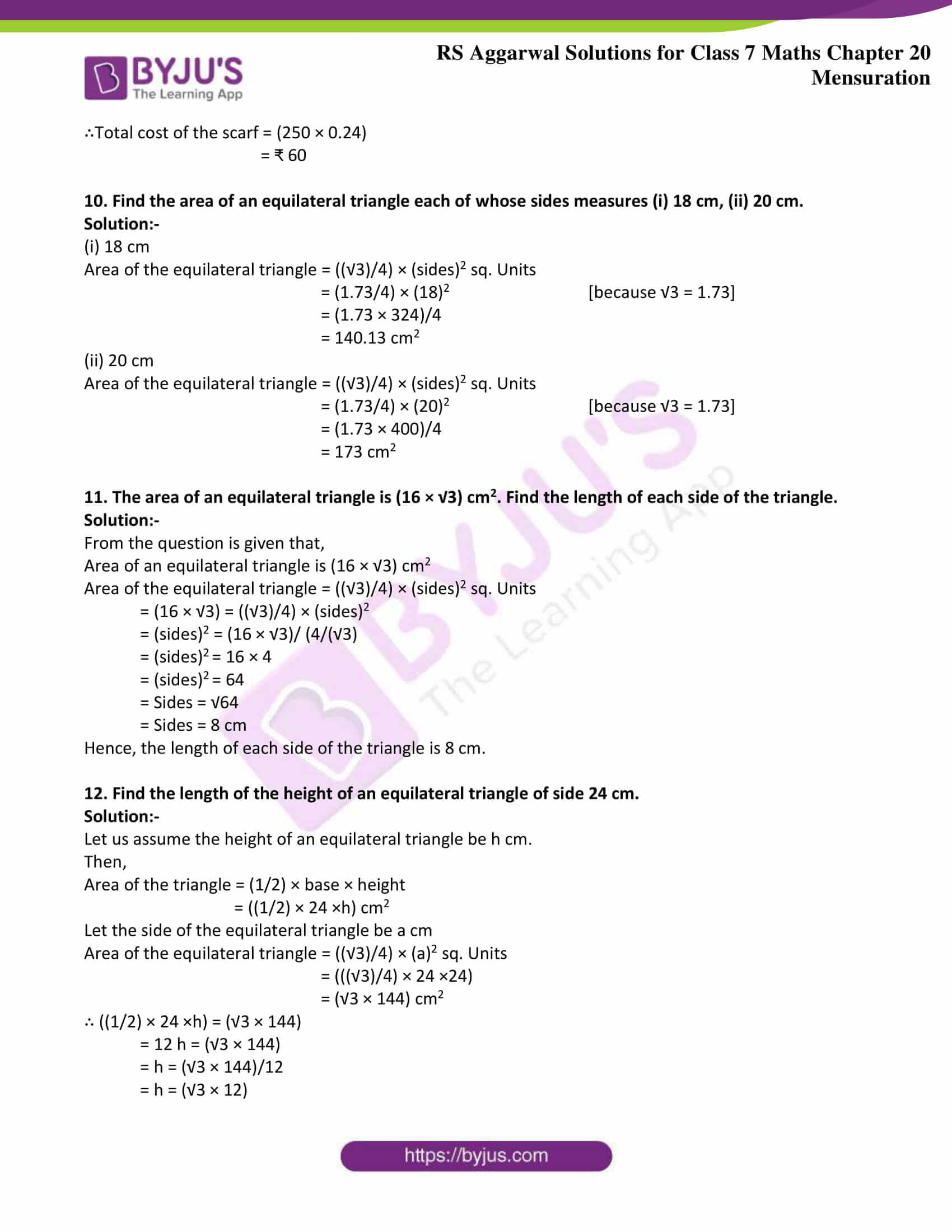### Access answers to Maths RS Aggarwal Solutions for Class 7 Chapter 20 – Mensuration Exercise 20D

1. Find the area of the triangle in which

(i) base = 42 cm and height = 25 cm.

Solution:-

Given,

base = 42 cm

height = 25 cm

∴ Area of the triangle = (1/2) × base × height

= (1/2) × 42 × 25

= 1 × 21 × 25

= 525 cm2

(ii) base = 16.8 m and height = 75 cm.

Solution:-

Convert the given measures into same units.

Given,

base = 16.8 m

height = 75 cm = (75/100) = 0.75 m [because 1 m = 100 cm]

∴ Area of the triangle = (1/2) × base × height

= (1/2) × 16.8 × 0.75

= 0.5 × 16.8 × 0.75

= 6.3 m2

(ii) base = 8 dm and height = 35 cm.

Solution:-

Convert the given measures into same units.

Given,

base = 8 dm = 8 × 10 = 80 cm [because 1 dm = 10 cm]

height = 35 cm

∴ Area of the triangle = (1/2) × base × height

= (1/2) × 80 × 35

= 1 × 40 × 35

= 1400 cm2

2. Find the height of the triangle having an area of 72 cm2 and base 16 cm.

Solution:-

From the question is given that,

Area of the triangle = 72 cm2

Base of the triangle = 16 cm

∴ Area of the triangle = (1/2) × base × height

72 = (1/2) × 16 × height

72 = 1 × 8 × height

Height = 72/8

Height = 9 cm

Hence, the height of the triangle is 9 cm.

3. Find the height of the triangular region having an area of 224 m2 and base 28 m.

Solution:-

From the question is given that,

Area of the triangle = 224 m2

Base of the triangle = 28 m

∴ Area of the triangle = (1/2) × base × height

224 = (1/2) × 28 × height

224 = 1 × 14 × height

Height = 224/14

Height = 16 m

Hence, the height of the triangle is 16 m.

4. Find the base of a triangle whose area is 90 cm2 and height 12 cm.

Solution:-

From the question is given that,

Area of the triangle = 90 cm2

Height of the triangle = 12 cm

∴ Area of the triangle = (1/2) × base × height

90 = (1/2) × base × 12

90 = 1 × 6 × base

Base = 90/6

Base = 15 cm

Hence, the base of the triangle is 15 cm.

5. The base of a triangular field is three times its height. If the cost of cultivating the field at ₹ 1080 per hectare is ₹ 14580, find its base and height.

Solution:-

Total cost of cultivating the field = ₹ 14580

Rate of cultivating the field = ₹ 1080 per hectares

Area of the field = (total cost/rate per hectares)

= (14580/1080)

= 13.5 hectares

= (13.5 × 10000) m2

= 135000 m2

Let the height of the field be x m.

Then, its base = 3x m

Area of the field = (1/2) × (3x) × (x)

= (3x2/2) m2

∴ (3x2/2) = 135000

= x2 = 135000 × (2/3)

= x2 = 90000

= x = √90000

= x = 300

Hence, the height of the field = 300m and its base is 3x = 3 × 300 = 900m

6. The area of the right triangular region is 129.5 cm2. If one of the sides containing the right angle is 14.8 cm, find the other one.

Solution:-

Let the length of the other side be h cm.

Then, area of the triangle = (1/2) × base × height

129.5 = (1/2) × 14.8 × h

129.5 = 1 × 7.4 × h

129.5 = 7.4h

h = 129.5/7.4

h = 17.5 cm

Hence, the length of the other side is 17.5 cm

7. Find the area of a right triangle whose base is 1.2 m and hypotenuse 3.7 m.

Solution:-

Given,

Base = 1.2 m

Hypotenuse = 3. 7 m

Height =?

By Pythagoras theorem, we have

Hypotenuse2 = Base2 + Height2

Height2 = Hypotenuse2 – Base2

Height2 = 3.72 – 1.22

Height2 = 13.69 – 1.44

Height2 = 12.25

Height = √12.25

Height = 3.5 m

Then,

Area of right triangle = (1/2) × base × height

= (1/2) × 1.2 × 3.5

= 0.5 × 1.2 × 3.5

= 2.1 m2

8. The legs of a right triangle are in the ratio 3: 4 and its area is 1014 cm2. Find the lengths of its legs.

Solution:-

Let the legs of the right triangle be 3x and 4x respectively

3x be the base of the right triangle

4x be the height of the right triangle

Then, area of the triangle = (1/2) × base × height

1014 = (1/2) × (3x) × (4x)

1014 = 0.5 × 12x2

1014 = 6x2

X2 = (1014/6)

X2 = 169

X = √169

X = 13

∴The base of the right triangle 3x = 3 × 13 = 39 cm

The height of the right triangle 4x = 4 × 13 = 52 cm

9. One side of a right-angled triangular scarf is 80 cm and its longest side is 1 m. Find its cost at the rate of ₹ 250 per m2.

Solution:-

Let us assume right angle triangular scarf ABC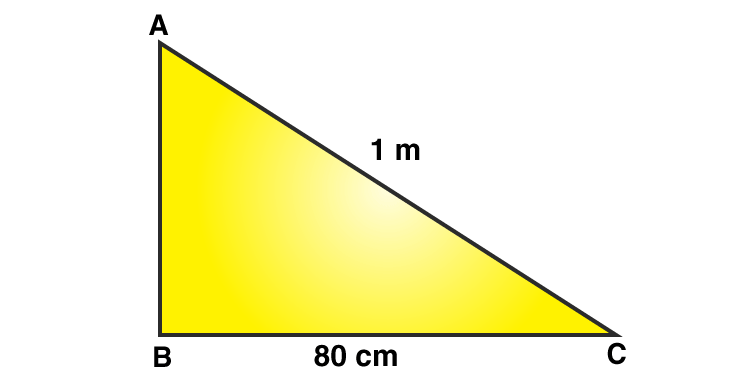Here, ∠B = 90o

BC = 80 cm

AC = 1 m = 100 cm

By Pythagoras theorem, we have

AC2 = BC2 + AB2

AB2 = AC2 – BC2

AB2 = 1002 – 802

AB2 = 10000 – 6400

AB2 = 3600

AB = √3600

AB = 60

Height = 60 cm

Then,

Area of the right-angled triangular scarf = (1/2) × BC × AB

= (1/2) × 80 × 60

= 1 × 40 × 60

= 2400 cm2

= 0.24 [Because 1 m2 = 10000 cm2]

Rate of the cloth ₹ 250 per m2

∴Total cost of the scarf = (250 × 0.24)

= ₹ 60

10. Find the area of an equilateral triangle each of whose sides measures (i) 18 cm, (ii) 20 cm.

Solution:-

(i) 18 cm

Area of the equilateral triangle = ((√3)/4) × (sides)2 sq. Units

= (1.73/4) × (18)2 [because √3 = 1.73]

= (1.73 × 324)/4

= 140.13 cm2

(ii) 20 cm

Area of the equilateral triangle = ((√3)/4) × (sides)2 sq. Units

= (1.73/4) × (20)2 [because √3 = 1.73]

= (1.73 × 400)/4

= 173 cm2

11. The area of an equilateral triangle is (16 × √3) cm2. Find the length of each side of the triangle.

Solution:-

From the question is given that,

Area of an equilateral triangle is (16 × √3) cm2

Area of the equilateral triangle = ((√3)/4) × (sides)2 sq. Units

= (16 × √3) = ((√3)/4) × (sides)2

= (sides)2 = (16 × √3)/ (4/(√3)

= (sides)2 = 16 × 4

= (sides)2 = 64

= Sides = √64

= Sides = 8 cm

Hence, the length of each side of the triangle is 8 cm.

12. Find the length of the height of an equilateral triangle of side 24 cm.

Solution:-

Let us assume the height of an equilateral triangle be h cm.

Then,

Area of the triangle = (1/2) × base × height

= ((1/2) × 24 ×h) cm2

Let the side of the equilateral triangle be a cm

Area of the equilateral triangle = ((√3)/4) × (a)2 sq. Units

= (((√3)/4) × 24 ×24)

= (√3 × 144) cm2

∴ ((1/2) × 24 ×h) = (√3 × 144)

= 12 h = (√3 × 144)

= h = (√3 × 144)/12

= h = (√3 × 12)

= h = 1.73 × 12

= h = 20.76 cm

Hence, the height of an equilateral triangle is 20.76 cm

### Access other exercises of RS Aggarwal Solutions For Class 7 Chapter 20 – Mensuration

Exercise 20A Solutions

Exercise 20B Solutions

Exercise 20C Solutions

Exercise 20E Solutions

Exercise 20F Solutions

Exercise 20G Solutions